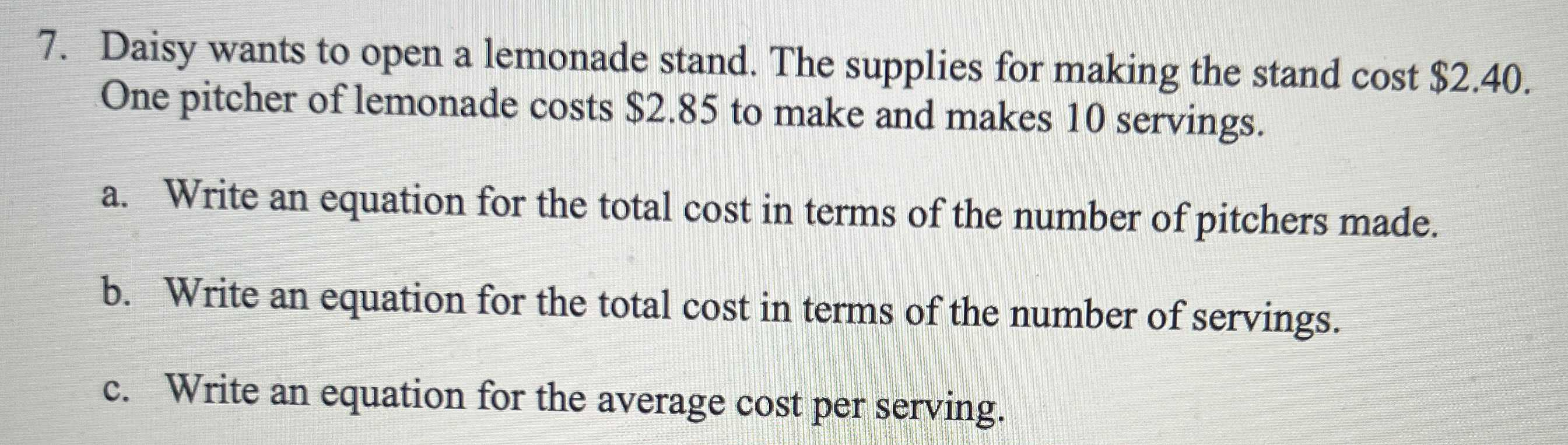### ¿Todavía tienes preguntas de matemáticas?

Pregunte a nuestros tutores expertos
Algebra
Pregunta7. Daisy wants to open a lemonade stand. The supplies for making the stand cost $$\ 2.40$$ . One pitcher of lemonade costs $$\ 2.85$$ to make and makes $$10$$ servings.

a. Write an equation for the total cost in terms of the number of pitchers made.

b. Write an equation for the total cost in terms of the number of servings.

c. Write an equation for the average cost per serving.

a. Let y be the cost and x be the no.of pitchers, therefore the equation looks like

y = 2.85x

b. Let y be the cost and x be the no.of servings , therefore the equation looks like

y = 0.285x

c. Let y be the average cost and x be the no.of servings , therefore the equation looks like

y = 2.85/x

Solución
View full explanation on CameraMath App.